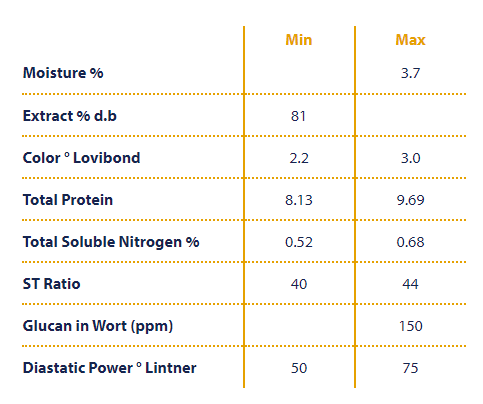# Beer Math—Working With Percentages and Gravity Units

Last month we looked at how to convert a recipe from grain percentages into a weight for whatever batch size you need, using the following formula.

This month, instead of that, we’ll look at how to use gravity units, or GU. GU is simply the digits after the decimal place of your specific gravity, multiplied by 1000. For example, a wort with an SG of 1.046 would have 46 gravity points.

Before you can convert a recipe from percentages to weights, some information is needed. Most can be found in the recipe, while the rest may require a little extra effort. Let’s use the simple one grain grist we had last month as a starting point.

Style – IPA
OG – 1.060
Efficiency – 72%
Volume – 20L
Ingredients – Simpsons Maris Otter – 100%

Before we can figure out how much malt we actually need, we need to figure out how many total gravity points this recipe has, and for that we’ll use the following formula.

Now that we know the total gravity points of the recipe, we need to know the potential of the grain – that is, how much sugar can we get out of the grain. You can find this in most brewing software, the BeerSmith website (http://beersmith.com/Grains/Grains/GrainList.htm), or from malt specification sheets/websites. If we look at the Simpsons website (https://www.simpsonsmalt.co.uk/our-malts/finest-pale-ale-maris-otter/), we get the following table that we saw last month.While this table doesn’t tell us the potential in GU, it does give it to us Extract % d.b. To convert that to GU we need to revisit a number from earlier, 46, and a concept you may have come across before, PPG (points/pound/gallon). Sucrose (white sugar) is the standard for sugar solutions, and when one pound of sucrose is dissolved into one gallon, we get an SG of 1.046, or 46 GU. We can then compare the Extract % d.b (%Extract cg, db from last month) of our grain to sucrose to get the grain’s potential as follows.

To get a more accurate potential, we could also factor the moisture content of the grain into it, but this is actually often ignored in various software and online calculators as the potential is typically rounded to the nearest whole number.

Now that we know the total points of the recipe, and the potential of the grain we can calculate how many kg of Maris Otter we need. There’s some new terms here as well as some from last month, so let’s go through them.

GW – grain weight
Total Points – total gravity points of the recipe
Potential – potential of the grain, expressed as GU
8.34 – this is our conversion factor to go from PPG to points/kg/L as we’re using litres for our batch size, and kilograms for our grain weight. (1 PPG = 1 point / 0.454 kg / 3.785 L=8.34 points/kg/L)
BH eff% – brewhouse efficiency expressed as a percentage

This formula is less accurate than the one used last month, and will actually give us a different answer. Last month’s formula gave us 5.68 kg, so a difference of 5%.

To take this one step further, we’ll take our four grain recipe from last month and do the calculations.

Style – IPA
OG – 1.060
Efficiency – 72%
Volume – 20L
IngredientsFor this recipe, I’ll use all Maker’s Malt from Rosthern, SK (https://makersmalt.com/) with the necessary grain information listed after.
Pale malt – 80% (%extract = 82.3%)
Munich 10 – 10% (%extract = 81.7%)
White wheat malt – 5% (%extract = 83.1%)
Caramel 60 – 5% (%extract = 81.0%)

For this, we’ll calculate the total points that each grain will contribute to the recipe by multiplying the Total Points by the percentages and update the potential for each grain based off the %extract, both listed above.

It’s important to note that there are different versions of these formulas out there, including those found in various brewing software, all with slight variations but they should all give you results that are very similar.

One common use of GU is to determine how much DME (dry malt extract) you can use to increase the gravity of your wort post-boil if you missed your target OG. Let’s assume our IPA had a post-boil SG of 1.057 but we wanted 1.060, and we want to increase our OG with DME which has a potential of 44.

For this, we’ll start off by calculating what our desired Total Points are. Here, the GU will be the difference between our targeted and actual OG.

Next, we’ll calculate the weight of our DME by using the grain weight formula, but we’ll ignore the efficiency (or just make it 100%) as this is a ready to use product.

So, to increase the gravity of our IPA from 1.057 to the targeted 1.060, we’ll need to add 0.16 kg of DME.

Next month, we’ll look at hops, how to calculate IBUs, and a better way to determine the bitterness of your beer with GU.